# STUDY ON EVOLUTION CHARACTERISTICS OF ISOTROPIC TURBULENCE PASSING THROUGH A NORMAL SHOCK WAVE1)

Hong Zheng2), Ye Zhengyin

School of Aeronautics, Northwestern Polytechnical University, Xi'an 710072, China

2) 洪正,硕士研究生,主要研究方向：激波湍流相互干扰. E-mail: hongzheng@mail.nwpu.edu.cn

Abstract

Shock-turbulence interaction is a kind of important fundamental problem in aerodynamics. Based on solver Helios which applies cell-centered finite difference method (CCFDM), using fifth-order weighted compact nonlinear scheme (WCNS), we conducted direct numerical simulation (DNS) of the situation where isotropic turbulence passes through a normal shock wave. Turbulence statistics are calculated for analysis. We found after shock, density is a little lower than its non-turbulent value, so do temperature and pressure, on the contrary, longitudinal velocity is a little higher than its non-turbulent value. The commonality is that they all show an overshoot immediately behind the shock, after that they gradually approach towards their non-turbulent values along with downstream distance. Longitudinal Reynolds stress suffers a sudden decrease and increases rapidly followed by decaying. This evolution characteristics is captured in linear interaction analysis (LIA) and a transfer of energy from acoustical to vertical modes behind the shock is thought to be accounted for it according to this analysis. Different from longitudinal Reynolds stress, Transverse Reynolds stress suffers a sudden increase then decay monotonically. Anisotropy of Reynolds stress is apparent after shock, and it gradually increases as downstream distance increases. Turbulent kinetic energy suddenly increases and then evolves non-monotonically. Taylor microscale and Kolmogorov scales apparently decrease after shock, indicating the decrease of turbulent length scales, which leads to a requirement of higher resolution of mesh in this zone to solve the flow field. After shock, the root-mean-squares of density, temperature and pressure fluctuations are enhanced, and intensities of density and pressure decrease while intensity of temperature increases.

Keywords： shock-turbulence interaction ; cell-centered finite difference method ; direct numerical simulation ; statistics analysis

0

Hong Zheng, Ye Zhengyin. STUDY ON EVOLUTION CHARACTERISTICS OF ISOTROPIC TURBULENCE PASSING THROUGH A NORMAL SHOCK WAVE1)[J]. Acta Mechanica Sinica, 2018, 50(6): 1356-1367 https://doi.org/10.6052/0459-1879-18-129

## 引 言

Kovansznay发现对于可压缩湍流中的任意脉动可以分解为3种独立的线性波模型,分别为声波、熵波、涡波.基于Kovansznay的分解,Rinber[2-4]使用线性分析的方法对STI问题进行了理论分析,发展出描述该问题的理论模型,称之为线性相互作用分析(linear interaction analysis,LIA),LIA假设激波前湍流脉动相比于平均流动是个小扰动,所以非线性和黏性效应就可以从分析中去除,这样就可以单独分析不同波通过激波后的变化.LIA成功地预测了通过激波后湍动能的增大和湍流长度尺度的减小.Lele利用快速变形理论(rapid distortion theory,RDT)和气体动力学理论推导了在湍流中的激波跳跃关系式,分析表明在特定的静压升下,湍流中的激波速度比在层流中稍大,压缩性增强.

Ryu和Livescu在湍流马赫数$1.1 < M_{\rm s} <2.2$和泰勒雷诺数$10 < Re_\lambda <45$参数范围内进行了一系列数值试验.结果表明,Kolmogorov尺度与激波厚度的比值越大,计算结果越接近LIA结果,而比值增大是通过减小湍流马赫数实现的,说明LIA在低湍流马赫数下是可信的,即使上游雷诺数较低的情况.Livescu和Ryu尝试通过LIA代替直接求解激波以减小计算量,从而进行任意平均流马赫数下波后的研究,但是远离激波或非线性及黏性作用明显的区域,未出现期望的衰减.Quadros等[14-15]利用LIA和DNS数据具体研究了波后湍流能量通量,基于线性理论结果提出了新的限制器模型,发展了基于物理的湍动能输运方程模型,对比发现在一系列马赫数范围下模型预测结果与已有DNS结果符合良好.

Tian等[16-17]利用LIA跟DNS研究比较上游多组分流动跟单组分流动在这类问题中的差异,以此来分析密度变化在该问题中产生的效应.发现上游较强的密度变化会使得湍流过激波后的增强及湍流长度尺度的减小相比单组分流动更加显著,激波引起的湍流混合增强也得到了提升,波后混合非对称性更强.

Gao等将五阶WENO(weighted essential non-oscillatory)非线性格式和六阶中心差分线性格式混合,研究了标量场在不同施密特数下随各向同性湍流通过正激波后统计量的演化,并与时间衰减各向同性湍流中的情形进行对比.Vemula和Sinha发现现有的雷诺应力模型在经典的激波与各向同性湍流相互作用问题中预测误差很大,于是他们从物理的角度出发修正了雷诺应力输运方程,结果表明在一系列马赫数下与DNS结果较为符合.Boukharfane等使用高分辨率格式对不同来流马赫数下通过激波后湍流中被动标量的混合进行数值模拟,发现激波显著改变了被动标量场的拓扑结构,增强了标量混合,且随来流马赫数增大而愈发明显.

## 1 控制方程及数值方法

### 1.1 控制方程

DNS方法的实质是求解非定常的Navier-Stokes方程,不使用任何模型,直接对流动中的各种大小尺度流动进行求解,要求网格尺度与时间步长要与流动最小尺度相当,相比于雷诺平均(Reynolds-averaged,RANS)方法和大涡模拟(large eddy simulation,LES)方法,对计算资源提出了更高的要求.本文使用无量纲的Navier-Stokes方程以及理想气体方程

$$\frac{\partial \rho }{\partial t} + \frac{\partial }{\partial x_i}(\rho u_i ) = 0 (1)$$$$\frac{\partial }{\partial t}(\rho u_i ) + \frac{\partial}{\partial x_j }(\rho u_i u_j + p\delta _{ij} ) - \frac{\partial\tau _{ij} }{\partial x_j } = 0(2)$$$$\frac{\partial }{\partial t}(\rho E) + \frac{\partial }{\partial x_i }((\rho E + p)u_i ) - \frac{\partial }{\partial x_j }(u_i \tau_{ij} ) + \frac{\partial q_i }{\partial x_i } = 0(3)$$$$\frac{1}{\gamma }\rho T (4)$$

$$\tau _{ij} = \frac{\mu }{Re}\left(\frac{\partial u_i }{\partial x_j } + \frac{\partial u_j }{\partial x_i } -\frac{2}{3}\frac{\partial u_k }{\partial x_k }\right) \\ q_i = - \frac{\mu c_p }{(\gamma - 1)RePr }\frac{\partial T}{\partial x_i } \\ E = \frac{p}{\rho (\gamma - 1)} + \frac{1}{2}u_i u_i \\ \gamma = \frac{c_p }{c_v } = 1.4$$

$$Re = \frac{\rho _0 c_0 L}{\mu _0 }$$

$$Pr = \frac{\mu _0 c_p }{k_0 }$$

### 1.3 入口条件

\begin{equation}\label{eq5} \mathop f\limits^ \wedge (k_1 ,k_2 ,k_3 ) =\frac{1}{N_x N_y N_z }\sum\limits_{x,y,z} {f(x,y,z){\rm e}^{ -{\rm i}(k_1 x + k_2 y + k_3 z)}} \tag{5}\end{equation}

$$f(t,y,z) = \sum\limits_{k_1 ,k_2 ,k_3 } {\mathop f\limits^ \wedge } (k_1 ,k_2 ,k_3 ){\rm e}^{{\rm i}(k_1 U_1 t +k_2 y + k_3 z)} (1)$$

## 2 数值方法验证

### 2.1 时间衰减各向同性湍流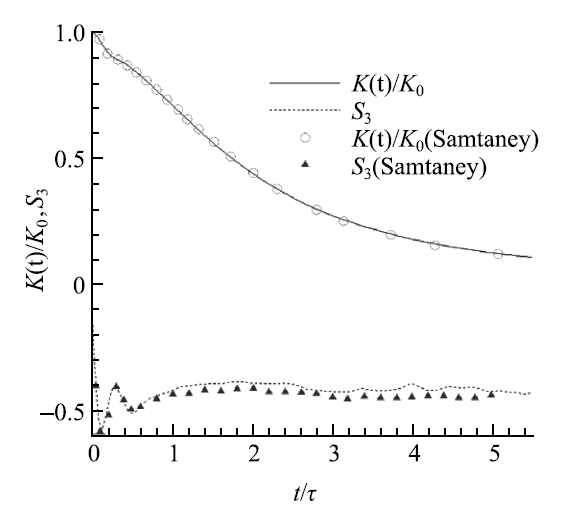Fig.1   Evolution of averaged turbulent kinetic energy and velocity derivative skewness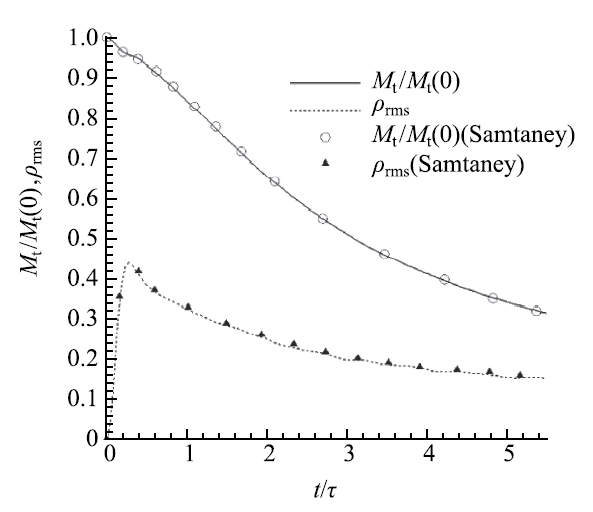Fig.2   Evolution of the turbulent Mach number and normalized root-mean-square density fluctuation

### 2.2 空间衰减各向同性湍流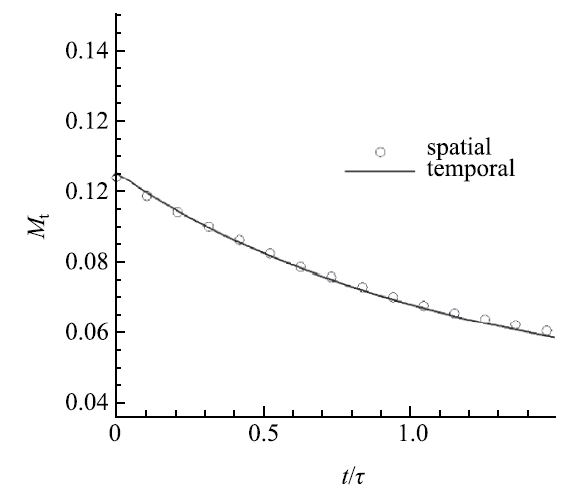Fig.3   Evolution of the turbulent Mach number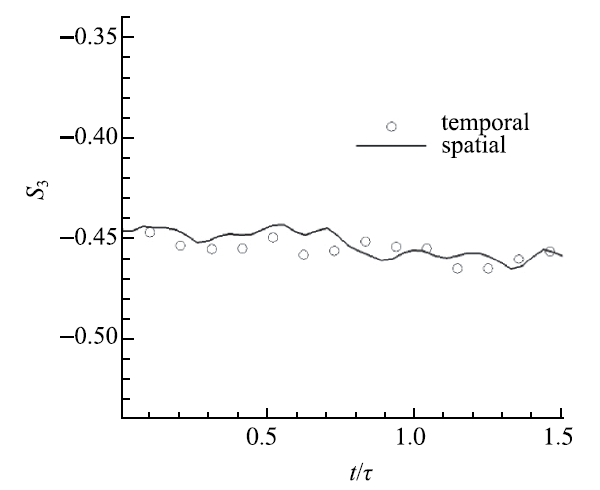Fig.4   Evolution of the velocity derivative skewness

### 2.3 网格收敛性验证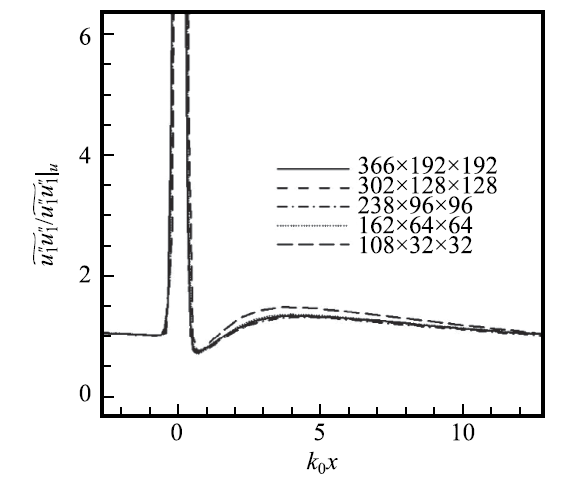Fig.5   Evolution of the longitudinal Reynolds stress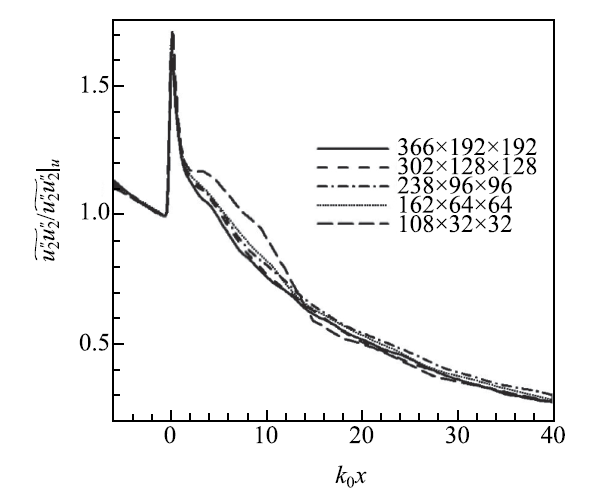Fig.6   Evolution of the transverse Reynolds stress

## 3 DNS结果及分析

### 3.1 计算模型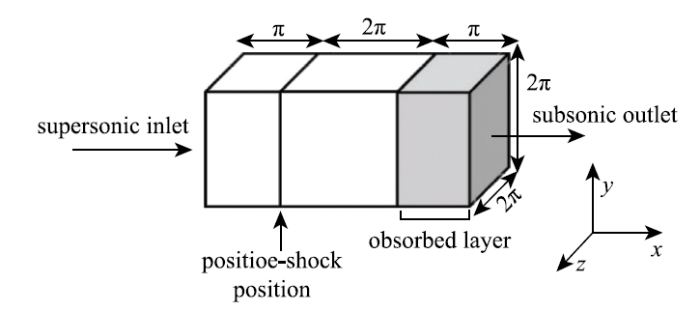Fig.7   The sketch of computational model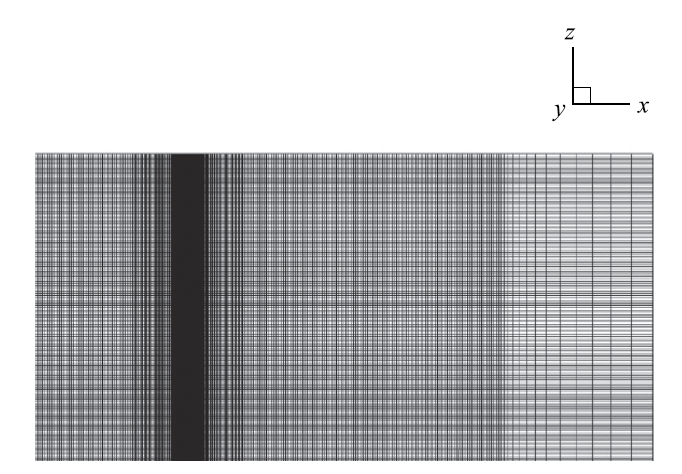Fig.8   Computational mesh

### 3.2 平均流场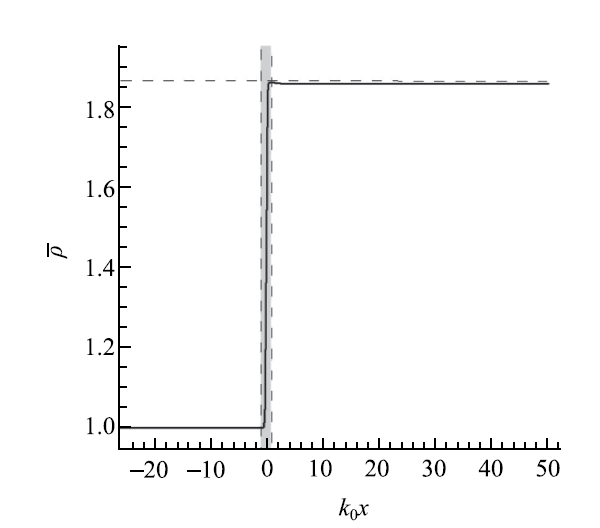Fig.9   Profile of the mean density (long-dash line denotes R-H value)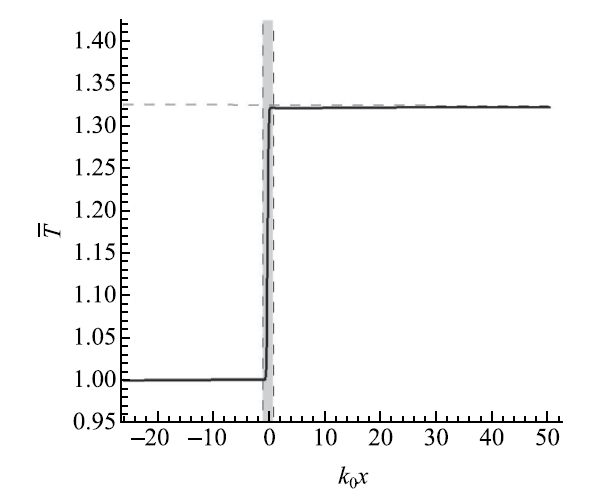Fig.10   Profile of the mean temperature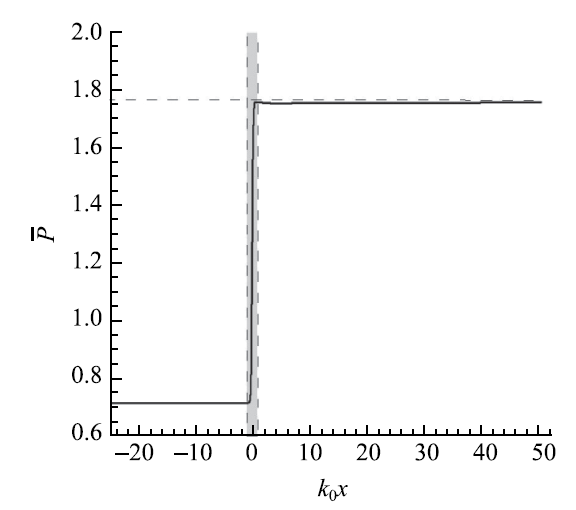Fig.11   Profile of the mean pressure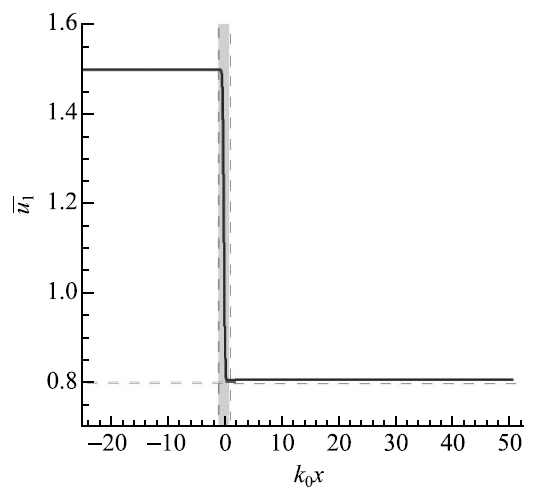Fig.12   Profile of the mean longitudinal velocity

Lele推导了湍流中的激波跳跃关系式,得到了湍流中激波过激波后压力增加较无湍流情况下变小的结论,这与数值的结果是一致的.

### 3.3 雷诺应力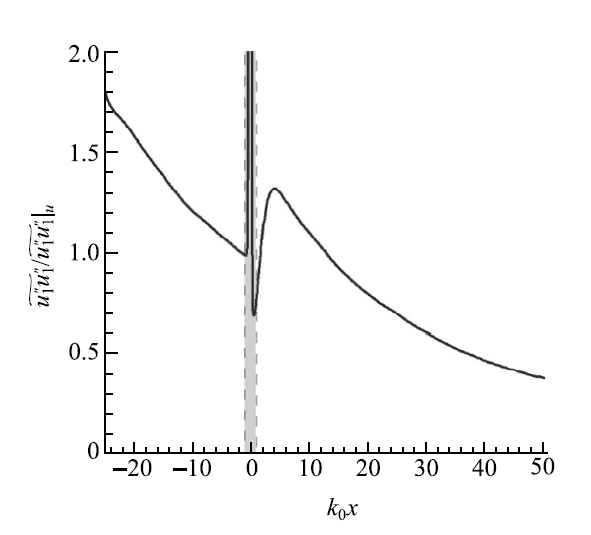Fig.13   Evolution of the longitudinal Reynolds stress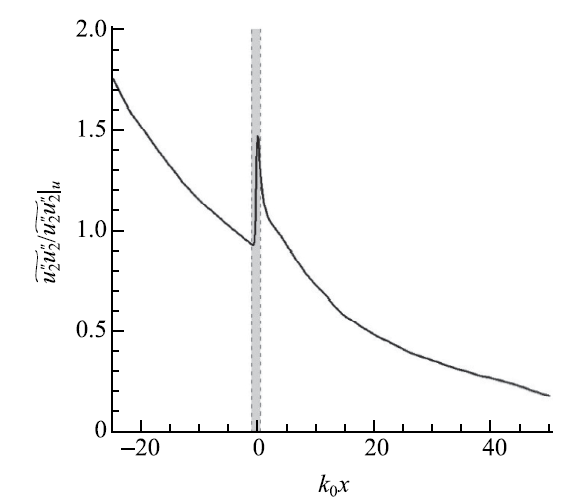Fig.14   Evolution of the transverse Reynolds stress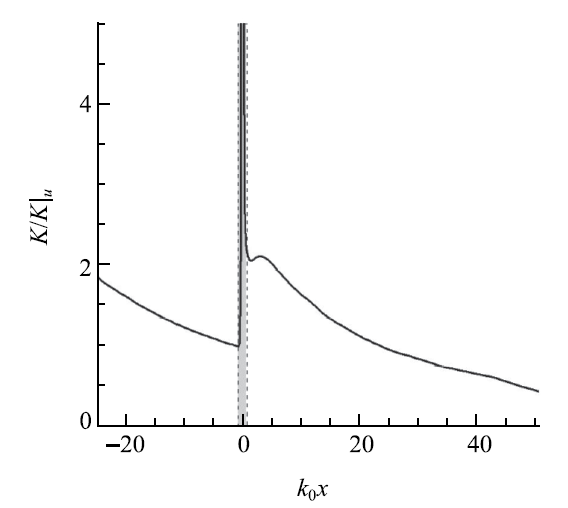Fig.15   Evolution of the turbulent kinetic energy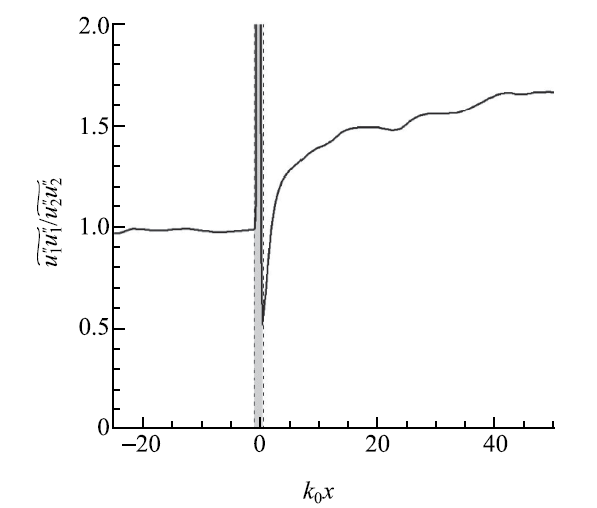Fig.16   Evolution of the ratio of longitudinal Reynolds stress to transverse one

### 3.4 涡量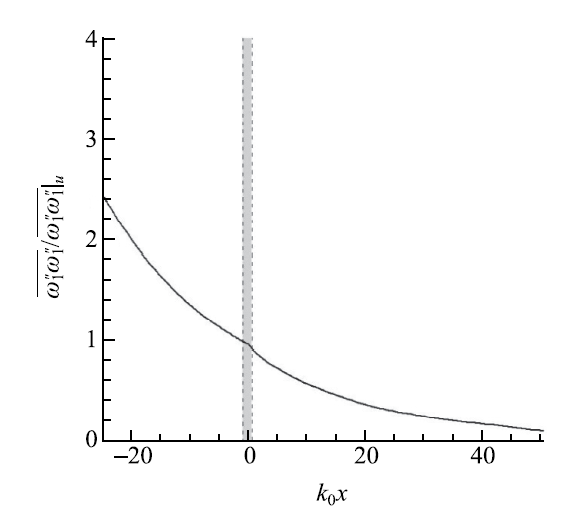Fig.17   Evolution of the longitudinal vorticity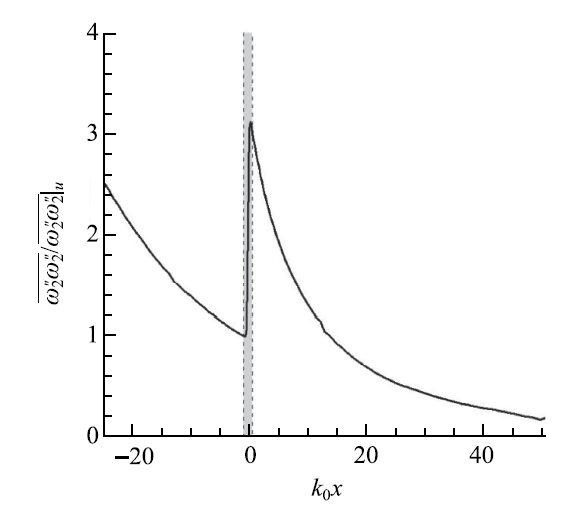Fig.18   Evolution of the transverse vorticity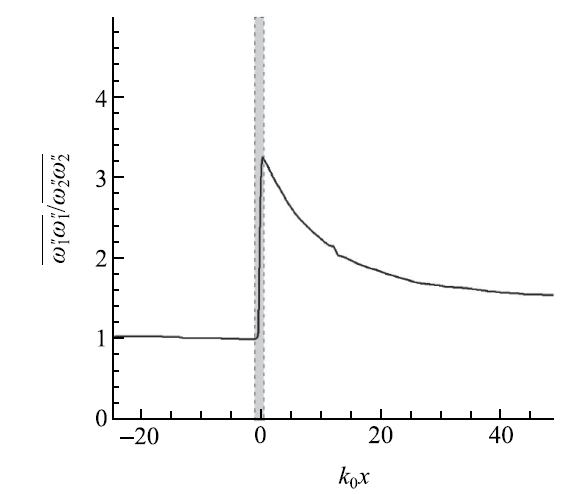Fig.19   Evolution of the ratio of longitudinal vorticity to transverse one

### 3.5 湍流长度尺度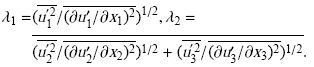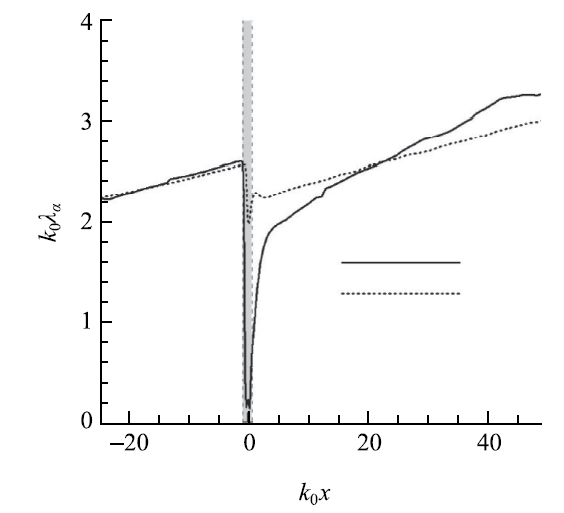Fig.20   Evolution of the longitudinal and transverse Taylor microscale

LIA分析得到的结果表明通过激波后$\lambda _1 < \lambda _2$,Lee等的DNS结果也呈现了相同的结论,但是Larsson网格分辨率、格式精度更高的DNS结果却表明,激波后$\lambda_1 < \lambda _2$,但是$\lambda _1$迅速增加以致短距离后$\lambda_1 > \lambda _2$.Larsson认为,与LIA结果相反的原因是由于在激波后泰勒微尺度的演化过程中非线性作用至关重要,而LIA忽略了非线性影响,Lee的结果由于网格分辨率不够,非线性的预测不足,所以更倾向于线性的结果.本文的结果是激波后距离约20内$\lambda _1 < \lambda _2$,之后$\lambda _1 > \lambda _2$,趋向于Larsson的结果.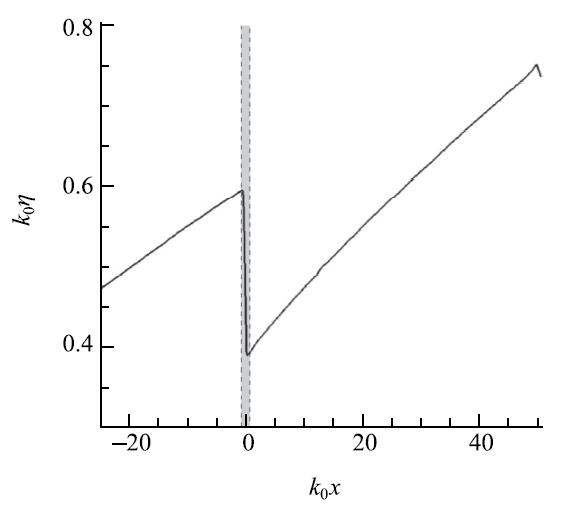Fig.21   Evolution of the Kolmogorov scale

### 3.6 热力学量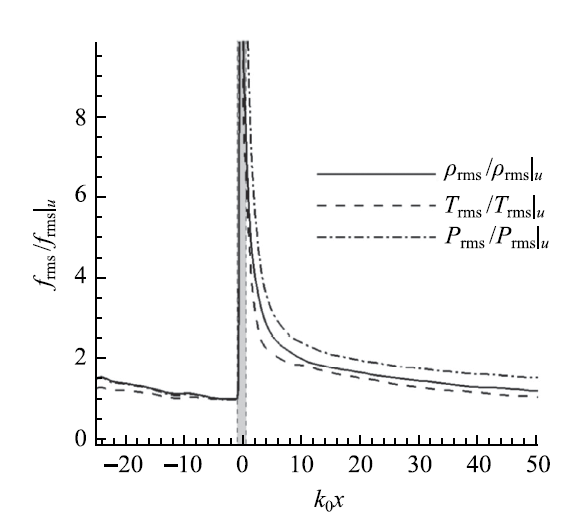Fig.22   Evolution of the root-mean-square fluctuation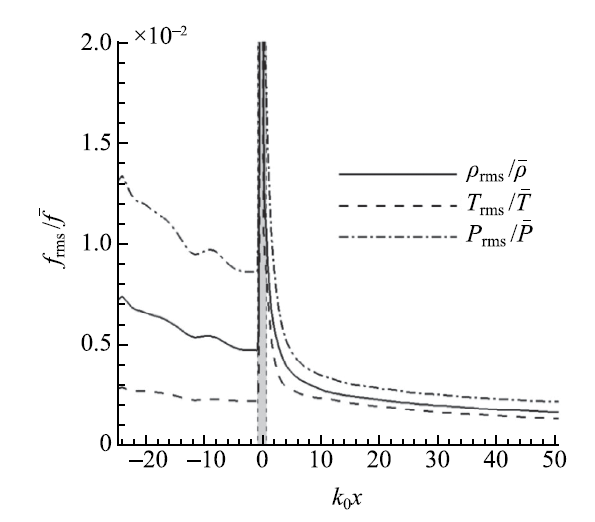Fig. 23   Evolution of the fluctuation intensity

### 3.7 流场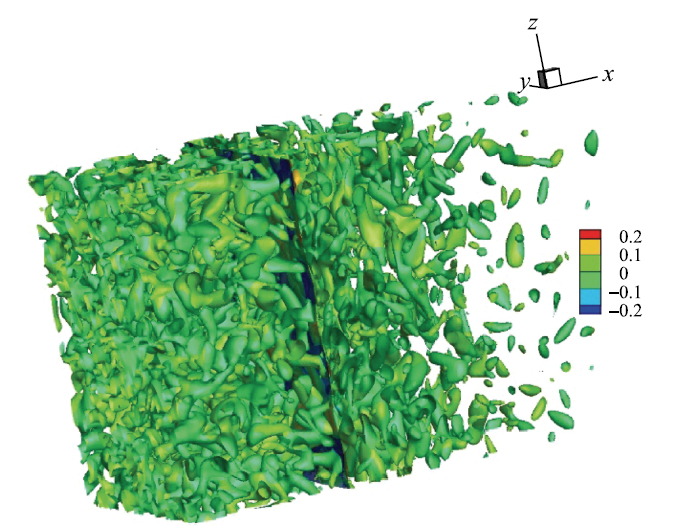Fig.24   Vortex structure characterized by Q criterion (dark plane represents the instantaneous shock surface, colored by pressure fluctuation)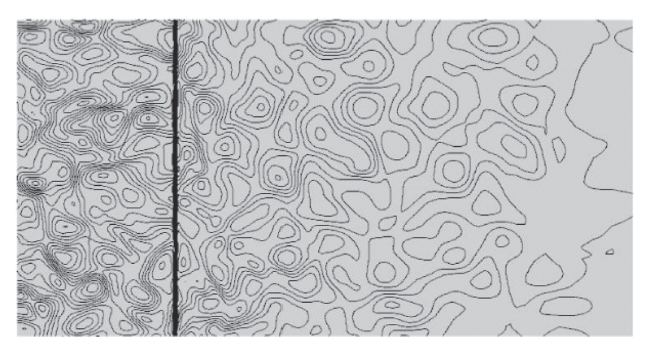Fig.25   Contour of fluctuation Mach number at $y=\pi$ plane

## 4 结 论

(1)同样的平均来流流动参数下,激波后的密度、温度和压力比无湍流情形下要低;波后的流向速度比无湍流情形下要高.

(2)过激波后,流向雷诺应力突降后快速达到峰值后衰减,横向雷诺应力突增后单调衰减.从流向与横向应力比值来看,激波后各向异性明显且随下游距离逐渐增强.湍动能波后得到增强,并呈现非单调的变化趋势.

(3)流向涡量在激波内有小幅上升随即衰减,激波前后没有明显变化;横向涡量波后明显的增强,呈现单调衰减的趋势.横向流向涡量的比值表明波后涡量的各向差异达到最大,然后随着下游距离逐渐减弱,但在本文计算中没有恢复到各向同性.

(4)波前各方向泰勒微尺度随空间位置逐渐增加,过激波后,流向横向均减小,流向减小得更多,随即快速增加,然后以较小速率继续随下游增大;横向尺度经过短暂的上升下降后,以与波前相同的速率随下游增加.波后$\lambda _1 < \lambda _2$,经过一段距离后则$\lambda _1 >\lambda _2$.Kolmogorov尺度过激波后减少约1/3,故对波后网格的分辨率提出了更高的要求.

(5)密度、温度和压力脉动均方根在穿过激波后均得到了增强;从脉动强度来看,密度和压力脉动强度波后减小,温度脉动强度增大,波前各脉动强度差异明显,波后却趋于一致.

The authors have declared that no competing interests exist.

## 参考文献 原文顺序 文献年度倒序 文中引用次数倒序 被引期刊影响因子

  Leslie SG, Kovasznay. Turbulence in supersonic flow. Journal of the Aeronautical Sciences, 1953, 20: 657-682  Ribner HS.Convection of a pattern of vorticity through a shock wave. NACA TN 2864, 1953  Ribner HS.Shock/turbulence interaction and the generation of noise. NACA TN 3288, 1954  Ribner HS.Spectra of noise and amplified turbulence emanating from shock/turbulence interaction. AIAA Journal, 1987, 35: 436-442  Lele SK.Shock-jump relations in a turbulent flow. Physics of Fluids A : Fluid Dynamics, 1992, 4: 2900-2905  Lee S, Lele SK, Moin P.Direct numerical simulation of isotropic turbulence interacting with a weak shock wave. Journal of Fluid Mechanics, 1993, 251: 533-562  Lee S, Lele SK, Moin P.Interaction of isotropic turbulence with shock waves: effect of shock strength. Journal of Fluid Mechanics, 1997, 340: 225-247  Mahesh K, Lele SK, Moin P.The influence of entropy fluctuations on the interaction of turbulence with shock wave. Journal of Fluid Mechanics, 1997, 334: 353-379  Lele SK, Larsson J.Shock-turbulence interaction: what we know and what we can learn from peta-scale simulations. Journal of Physics: Conference Series, 2009, 180: 012032  Larsson J, Lele SK.Direct numerical simulation of canonical shock/turbulence interaction. Physics of Fluids, 2009, 21: 126101  Larsson J, Bermejo-Moreno I, Lele SK.Reynolds- and Machnumber effects in canonical shock-turbulence interaction. Journal of Fluid Mechanics, 2013, 717: 293-321  Ryu J, Livescu D.Turbulence structure behind the shock in canonical shock-vortical turbulence interaction. Journal of Fluid Mechanics, 2014, 756: R1  Livescu D, Ryu J.Vortitity dynamics after the shock-turbulence interaction. Shock Waves, 2016, 26: 241-251  Quadros R, Sinha K, Larsson J.Turbulent energy flux generated by shock/homogeneous-turbulence inter-action. Journal of Fluid Mechanics, 2016, 796: 113-157  Quadros R, Sinha K.Modeling of turbulent energy flux in canonical shock-turbulence interaction. International Journal of Heat and Fluid Flow, 2016, 61: 626-635  Tian YF, Jaberi FA, Livescu D, et al.Numerical simulation of multi-fluid shock-turbulence interaction. AIP Conference Proceedings, 2017, 1793:150010  Tian YF, Jaberi FA, Li ZR, et al.Numerical study of variable density turbulence interaction with a normal shock wave. Journal of Fluid Mechanics, 2017, 829: 551-588  Gao XY, Bermejo-Moreno I, Larsson J.Direct numerical simulation of passive scalar mixing in shock turbulence interaction //70th Annual Meeting of the APS Division of Fluid Dynamics, Denver, Colorado, 2017  Vemula JB, Sinha K.Reynolds stress models applied to canonical shock-turbulence interaction. Journal of Turbuulence, 2017, 18: 653-687  Boukharfane R, Bouali Z, Mura A.Evolution of scalar and velocity dynamics in planar shock-turbulence interaction. Shock Waves, 2018, 5: 1-25  王国蕾, 陆夕云. 激波和湍流相互作用的数值模拟.力学进展, 2012, 42(3): 274-281 (Wang Guolei, Lu Xiyun.Numerical simulation of shock wave/turbulence interactions. Advances in Mechanics, 2012, 42(3): 274-281(in Chinese))  崔光耀, 潘翀, 高琪等. 沟槽方向对湍流边界层流动结构影响的实验研究. 力学学报, 2017, 49(6): 1201-1212 (Cui Guanyao, Pan Chong, Gao Qi, et al.Flow structure in the turbulent boundary layer over directional riblets surfaces. Chinese Journal of Theoretical and Applied Mechanics, 2017, 49(6): 1201-1212(in Chinese))  童福林, 李欣, 于长平等. 高超声速激波湍流边界层干扰直接数值模拟研究. 力学学报, 2018, 50(2): 197-208 (Tong Fulin, Li Xin, Yu Changping, et al.Direct numerical simulation of hypersonic shock wave and turbulent boundary layer interactions. Chinese Journal of Theoretical and Applied Mechanics, 2018, 50(2): 197-208(in Chinese))  高天达, 孙姣, 范赢等. 基于PIV技术分析颗粒在湍流边界层中的行为. 力学学报, DOI: 10.6052/0459-1879-18-211 (PIV experimental investigation on the behavior of particle in the turbulent boundary layer. Chinese Journal of Theoretical and Applied Mechanics, DOI: 10.6052/0459-1879-18-211  Liao F, Ye ZY, Zhang LX.Extending geometric conservation law to cell-centered finite difference methods on stationary grids. Journal of Computational Physics, 2015, 284: 419-433  Liao F, Ye ZY.Extending geometric conservation law to cell-centered finite difference methods on moving and deforming grids. Journal of Computational Physics, 2015, 303: 212-221  Deng XG, Zhang HX.Developing high-order weigh-ted compact nonlinear schemes. Journal of Computational Physics, 2000, 165: 22-44  刘昕, 邓小刚, 毛枚良等. 高阶精度非线性格式WCNS-E-5在二维流动中的应用研究.空气动力学报, 2004, 22(2): 206-210 (Liu Xin, Deng Xiaogang, Mao Meiliang, et al.Weighted compact high-order nonlinear scheme WCNS-E-5 applied to two dimensional flows. Chinese Journal of Theoretical Applied Mechanics, 2004, 22(2): 206-210(in Chinese))  Mahesh K, Moin P, Lele SK.The interaction of a shock wave with a turbulent shear flow. AFSOR TF- 69, 1996  Rogallo RS.Numerical experiments in homogeneous turbulence. NASA, Technical Report 81315, 1981  秦泽聪, 方乐. 一种改进的均匀各向同性湍流初始化方法. 力学学报, 2016, 48(6): 1319-1325 (Qin Zecong, Fang Le.An improved method for initializing homogeneous isotropic turbulent flows. Chinese Journal of Theoretical and Applied Mechanics, 2016, 48(6): 1319-1325(in Chinese))  Samtaney R, Pullin DI, Kosovic B.Direct numerical simulation of decaying compressible turbulence and shocklet statistics. Physics of Fluids, 2001, 13: 1415-1430  Pope SB. Turbulent Flows.Cambridge: Cambridge University Press, 2000/

 〈〉